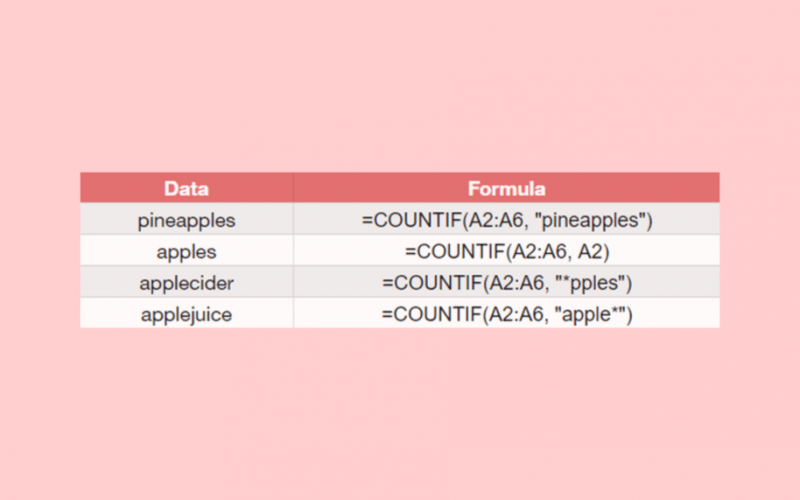# How to Use UNARY_PERCENT Function in Google Sheets

The UNARY_PERCENT function in Google Sheets is useful if you want to interpret a number into a percentage value.

The `UNARY_PERCENT` does this simply by combining the syntax together with a number, or if the number is in the cell, you can just click the specific cell.

You may not find the `UNARY_PERCENT` function necessary, but inevitably it comes handy, especially when working on complex or large data spreadsheets.

Let’s take an example to understand this function better.

Say, for example, you are in charge of preparing a budget for your department. The management allocated \$50,000 for your department’s needs, and you have to supply a budget report.

So how do we do that?

Simple. First, we identify our department’s necessities and arrange them according to priority. Then, we can use the `UNARY_PERCENT` function to convert the numbers into percentage values. Then, we will now multiply the percentage value with the said budget to arrive at a certain amount per need.

See how easy that is?

Another example that the `UNARY_PERCENT` function may be helpful is when we deal with financial and/or loan calculations using functions like `PMT`, `IPMT`, `PPMT`, and so on. We can have the `UNARY_PERCENT` nested in those formulas for you to arrive at a precise answer in an instant.

Let’s dive into real-business examples where we will deal with actual values and textual strings to appreciate the concept and how we can write our own `UNARY_PERCENT` function in Google Sheets to compute those data.

### The Anatomy of the UNARY_PERCENT Function

So the syntax (or the way we write) the UNARY function is as follows:

`=UNARY_PERCENT(percentage)`

Let’s break this down to understand better what each of the terms means:

• `=` the equal sign is just how we start any function in Google Sheets.
• `UNARY_PERCENT()` is our `UNARY_PERCENT` function where it will return a `PERCENTAGE` value.
• `PERCENTAGE` is the value that we want to interpret as a percentage (e.g. 20, 40, 100, or a selected cell in a sheet that contains a number)

### A Real Example of Using UNARY_PERCENT Function

Take a look at the example below to see how `UNARY_PERCENT` functions are used in Google Sheets.In the example above, the `UNARY_PERCENT` is used to convert the numbers in column D into percentage values. We then multiplied the allocated budget with the `UNARY_PERCENT` value to arrive at the allocated amount. Since our allocated budget of \$50,000 is constant, we made use of the dollar (\$) symbol to make it absolute.

`=\$B\$2*unary_percent(D2)`

Here’s what this example does:

• We have listed in column C as particulars all the marketing activities that our marketing department will take on. We also specified the percentage value for each activity, as seen in column D.
• Then, we have selected the cell E2 to make it an active cell. This is where we wrote our formula.
• We started our formula with an equal sign ‘=‘ followed by an opening parenthesis ‘(‘ and the cell B2.
• Since we wanted to make B2 absolute, we added a dollar sign (\$) before the letter B, and another dollar sign before the number 2 (\$B\$2).
• Now, we multiply \$B\$2 with the percentage value. Since the percentage in column D are presented in numbers, we, therefore, need to transform them by using `UNARY_PERCENT`.
• Close the formula with a close parenthesis ‘)‘, then hit on the Enter key.
• Make sure your formula should look like this: =\$B\$2*unary_percent(D2), before dragging it down to E7.

Super easy, right?

You may make a copy of the spreadsheet using the link I have attached below:

You can try it out by yourself.

Let’s begin writing our own UNARY_PERCENT function in Google Sheets.

### How to Use UNARY_PERCENT Function in Google Sheets

1. Simply click on an empty cell to make it active. This is where we will write our formula. For this guide, I selected the cell E2.1. Start your formula with an equal sign ‘=‘, just how we start any other function in Google Sheets.1. Next step, we will then select the cell B2. Since we will multiply this same amount with a percentage value, let’s make it absolute by adding a dollar sign (\$) before the letter B, and before the number 2.1. Then, we will use an asterisk sign (*). This denotes a multiplication operation.1. Since we want to multiply the allocated budget with a percentage value, and the percentage values in column D are presented in numbers, we will then convert them into percentage using our function. So, for the fifth step, we will write our function, followed by an open parenthesis ‘(‘. Wait for the auto pop-up message, as this will act as our extra guide in writing our formula.1. The numbers are already written in the cells found in column D. All we have to do is to just select them. In this step, we will select the cell D2.1. We will now close our formula with a close parenthesis ‘)‘. Then hit on the Enter key.1. Let’s now drag our formula until we reach the cell E7.That’s pretty much it. You can now use the UNARY_PERCENT function together with the other numerous Google Sheets formulas to create even more powerful formulas that can make your life much easier. 🙂

##### You May Also Like## How to ROUND Numbers in Google Sheets

The ROUND function in Google Sheets is useful if you want to reduce a value by a specific…## The Ultimate Guide to Using ISBLANK Functions in Google Sheets

The ISBLANK function is useful if you want to find out whether a cell is empty. Let’s take…## How to Get Subscript and Superscript Values in Google Sheets

A few days back I was making some changes and adding data into a Google Sheet as part…## How to Insert Excel Table in Gmail with Borders

Occasionally you’ll want to insert an Excel table directly into your Gmail. Besides the fact that it looks…